Courses

# General Organic Chemistry MCQ -1 (Advanced)

## 15 Questions MCQ Test Chemistry for JEE Advanced | General Organic Chemistry MCQ -1 (Advanced)

Description
This mock test of General Organic Chemistry MCQ -1 (Advanced) for JEE helps you for every JEE entrance exam. This contains 15 Multiple Choice Questions for JEE General Organic Chemistry MCQ -1 (Advanced) (mcq) to study with solutions a complete question bank. The solved questions answers in this General Organic Chemistry MCQ -1 (Advanced) quiz give you a good mix of easy questions and tough questions. JEE students definitely take this General Organic Chemistry MCQ -1 (Advanced) exercise for a better result in the exam. You can find other General Organic Chemistry MCQ -1 (Advanced) extra questions, long questions & short questions for JEE on EduRev as well by searching above.
QUESTION: 1

### Which of the following statements are incorrect with respect to the allotropes of carbon?  I) Diamond is extremely hard while graphite is soft  II) Diamond is ionic but graphite is covalent  III) Diamond is a non-conductor but graphite is a good conductor of electricity IV) Diamond has a layered structure while graphite has a network structure

Solution: Here the correct answer is C as in the question we can see that there is S. I. R effect so the c 1 and c5 bond lengths are of the same length and longer than the c3 bond length.... M.
*Multiple options can be correct
QUESTION: 2

### Choose the incorrect statement:

Solution:

In salicylic acid, there is H-bonding in conjugate base.

*Multiple options can be correct
QUESTION: 3

### In which of the following pairs the first one is the stronger base than second.

Solution: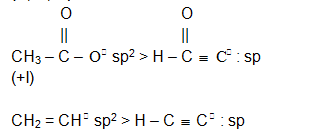*Multiple options can be correct
QUESTION: 4

Which statement among the followijng are corect?

Solution:
*Multiple options can be correct
QUESTION: 5

Resonance structures of a molecule should have.

Solution: Resonating structures must have identical arrangements of atoms, nearly same energy content and same number of unpaired electrons .
QUESTION: 6

Solution: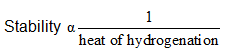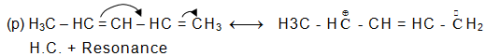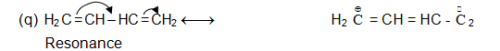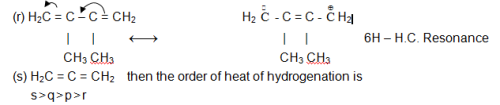QUESTION: 7

An organic compound on analysis gave C = 48 gm, H =  8 gm and N = 56 gm. Volume of 1.0 g of the compound was found to be 200 ml at NTP. Molecular formula of the compound is

Solution:
*Multiple options can be correct
QUESTION: 8

The most stable resonating structure of following compound is O =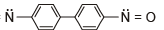Solution:

Maximum charge separation stability increases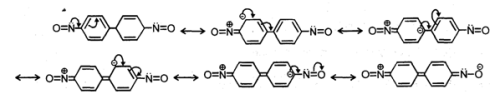Then and is (D).

*Multiple options can be correct
QUESTION: 9

2.34 The correct resonation structre of 1, 3-butadiene is -

Solution:
*Multiple options can be correct
QUESTION: 10

The correct stable resonating structure of benzene is -

Solution:
QUESTION: 11

Which resonating sturcture is not correct

Solution:
QUESTION: 12

Match the following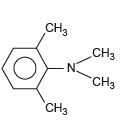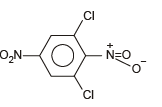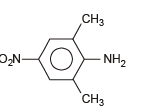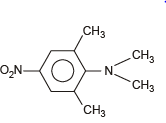p) m is more
(q) m is less
(r) Larger C– N compared to C – NO2
(s) Larger C – N bond largteer compared to aniline

Solution:

Stearic inhibition of resonance cause no change in bond length.

QUESTION: 13

Match the pKa values with the given compounds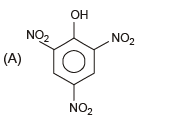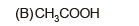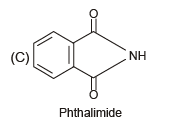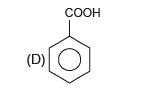(p) 4
(q) 9
(r) 5
(s) 0.74

Solution:

Remember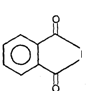NH is acidic instead of basic due to three resonating of  Conjugate base. As Shown in the adjacent diagram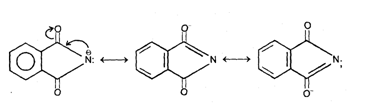It shows that conjugate base is very stable. The basic site in phthalimide is 0 not be N atom. Again it is due to resonance stabilisation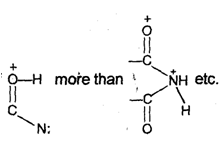QUESTION: 14

For the compound :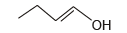Give the number

Solution:
QUESTION: 15

The number of sigma and pi-bonds in 1-butene 3-yne are

Solution: